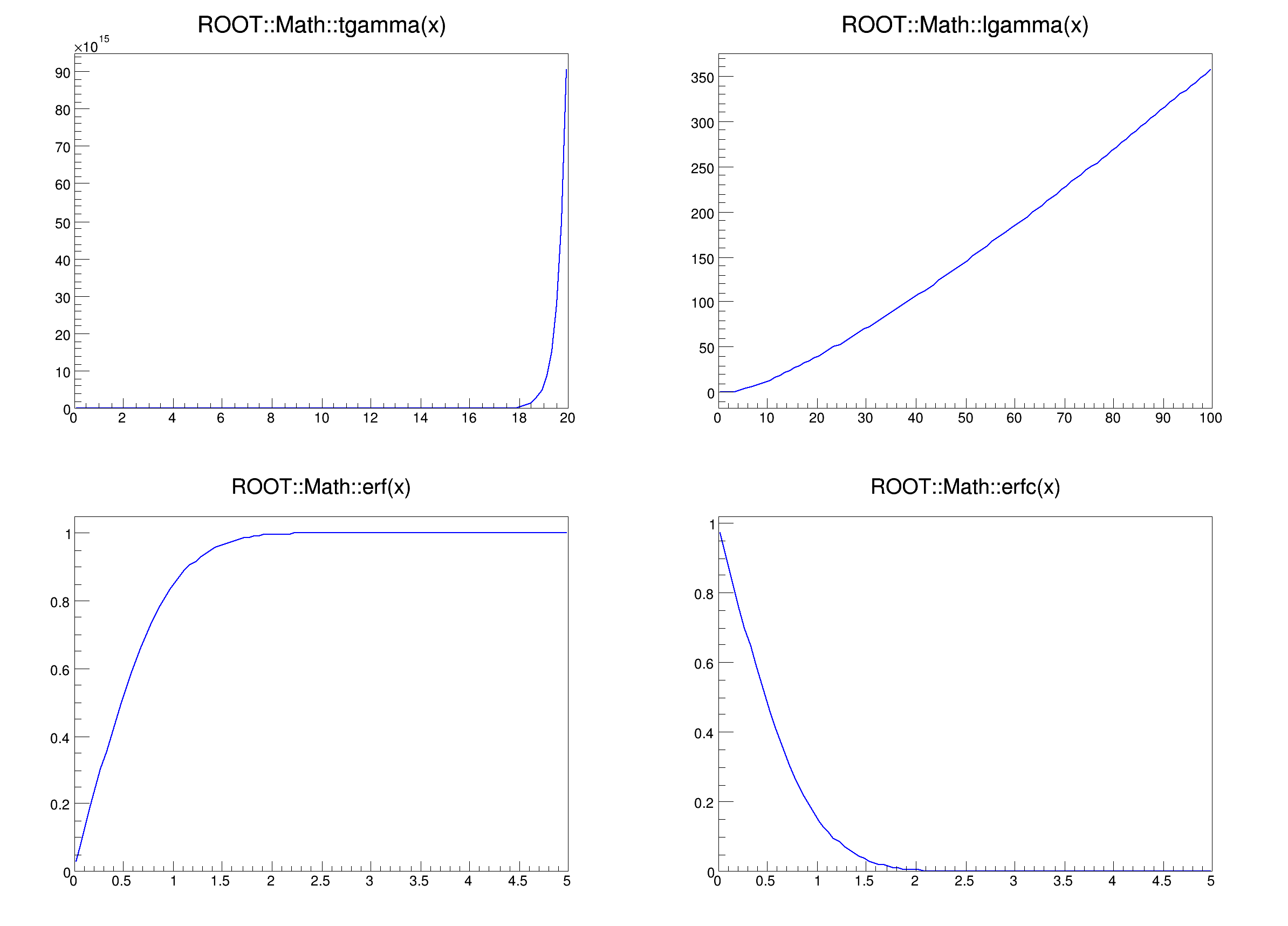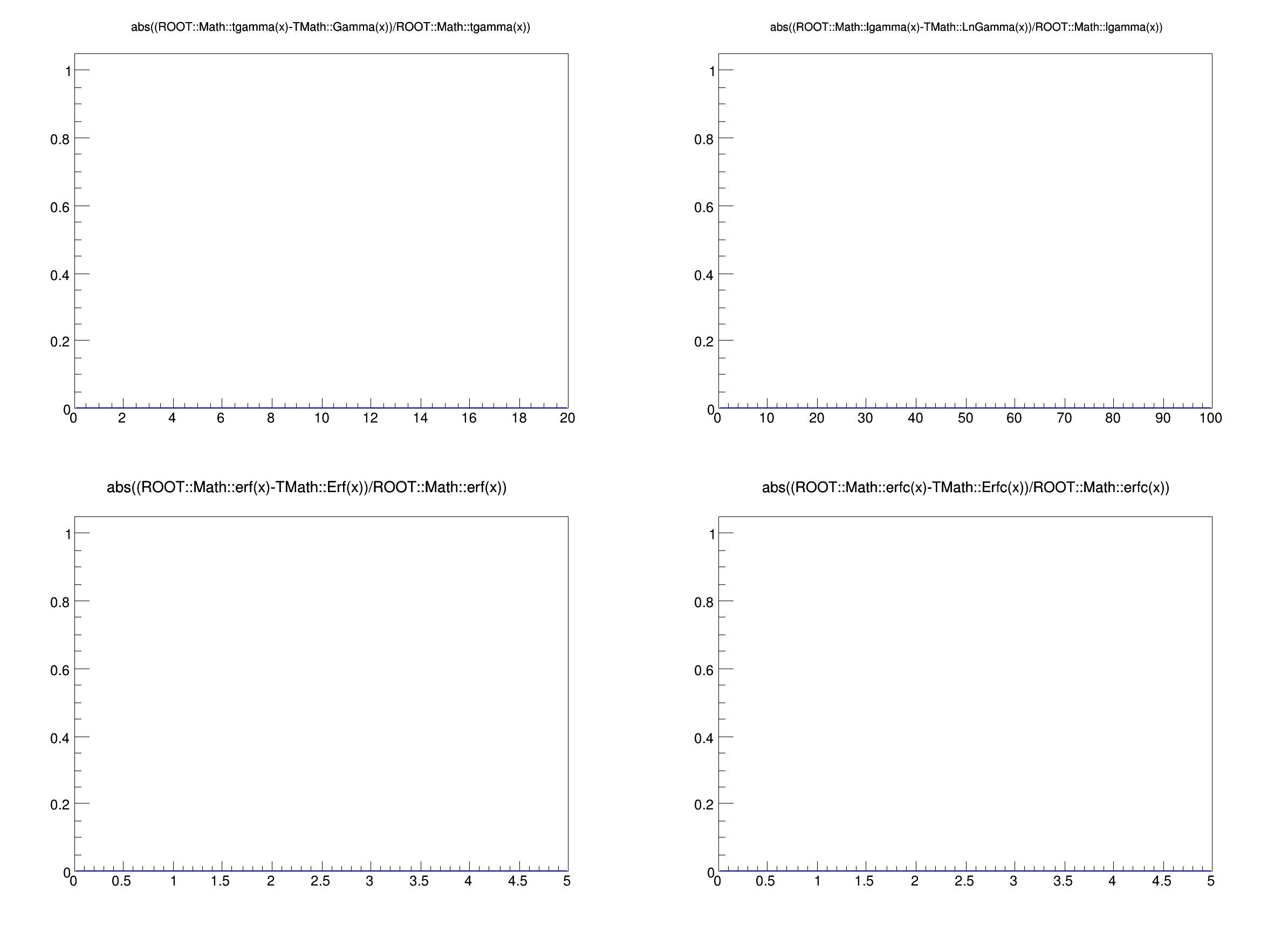ROOT   Reference GuidemathcoreSpecFunc.C File Reference

## Detailed DescriptionExample macro describing how to use the special mathematical functions taking full advantage of the precision and speed of the C99 compliant environments.

To execute the macro type in:

root .x mathcoreSpecFunc.C

It will create two canvases:

1. one with the representation of the tgamma, lgamma, erf and erfc functions
2. one with the relative difference between the old ROOT versions and the C99 implementation (on obsolete platform+compiler combinations which are not C99 compliant it will call the original ROOT implementations, hence the difference will be 0)

The naming and numbering of the functions is taken from [Matt Austern, (Draft) Technical Report on Standard Library Extensions, N1687=04-0127, September 10, 2004](A HREF="http://www.open-std.org/jtc1/sc22/wg21/docs/papers/2004/n1687.pdf")#include "TF1.h"
#include "TSystem.h"
#include "TCanvas.h"
void mathcoreSpecFunc() {
TF1 *f1a = new TF1("f1a","ROOT::Math::tgamma(x)",0,20);
TF1 *f1b = new TF1("f1b","abs((ROOT::Math::tgamma(x)-TMath::Gamma(x))/ROOT::Math::tgamma(x))",0,20);
TF1 *f2a = new TF1("f2a","ROOT::Math::lgamma(x)",0,100);
TF1 *f2b = new TF1("f2b","abs((ROOT::Math::lgamma(x)-TMath::LnGamma(x))/ROOT::Math::lgamma(x))",0,100);
TF1 *f3a = new TF1("f3a","ROOT::Math::erf(x)",0,5);
TF1 *f3b = new TF1("f3b","abs((ROOT::Math::erf(x)-TMath::Erf(x))/ROOT::Math::erf(x))",0,5);
TF1 *f4a = new TF1("f4a","ROOT::Math::erfc(x)",0,5);
TF1 *f4b = new TF1("f4b","abs((ROOT::Math::erfc(x)-TMath::Erfc(x))/ROOT::Math::erfc(x))",0,5);
TCanvas *c1 = new TCanvas("c1","c1",800,600);
c1->Divide(2,2);
c1->cd(1);
f1a->Draw();
c1->cd(2);
f2a->Draw();
c1->cd(3);
f3a->Draw();
c1->cd(4);
f4a->Draw();
TCanvas *c2 = new TCanvas("c2","c2",800,600);
c2->Divide(2,2);
c2->cd(1);
f1b->Draw();
c2->cd(2);
f2b->Draw();
c2->cd(3);
f3b->Draw();
c2->cd(4);
f4b->Draw();
}
@ kBlue
Definition: Rtypes.h:66
virtual void SetLineColor(Color_t lcolor)
Set the line color.
Definition: TAttLine.h:40
The Canvas class.
Definition: TCanvas.h:23
1-Dim function class
Definition: TF1.h:213
virtual void Draw(Option_t *option="")
Draw this function with its current attributes.
Definition: TF1.cxx:1322
return c1
Definition: legend1.C:41
return c2
Definition: legend2.C:14

Definition in file mathcoreSpecFunc.C.